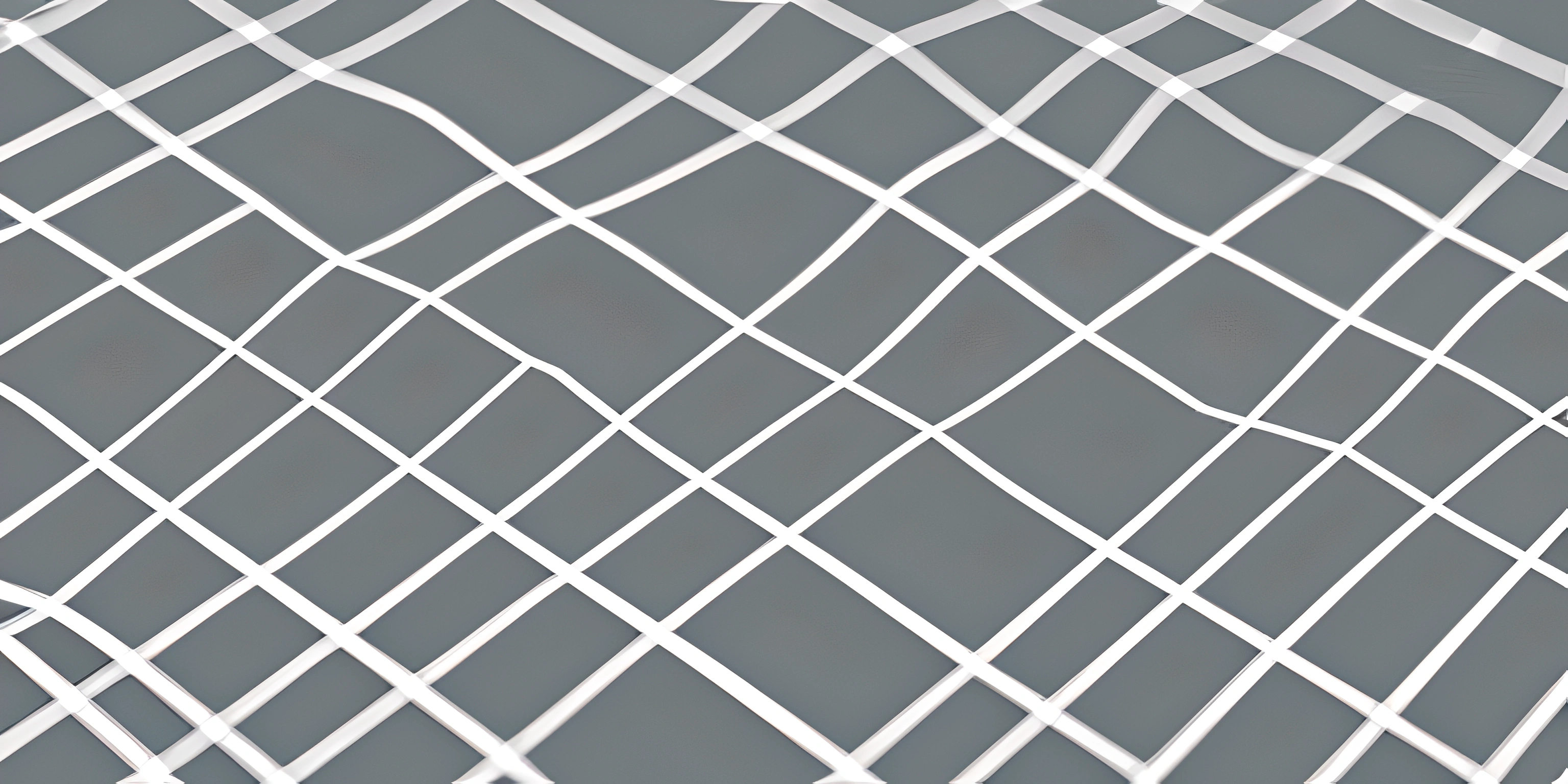# Pointers in CNote: this page has been created with the use of AI. Please take caution, and note that the content of this page does not necessarily reflect the opinion of Cratecode.

Pointers are a powerful feature in the C programming language that often intimidates newcomers. But fear not! We'll unravel the mystery of pointers and show you how to tame this wild beast.

## What is a Pointer?

A pointer is a variable that stores the memory address of another variable or value. It's like a treasure map to the location of another piece of data, except there be no pirates here, just happy programmers!

### Declaring Pointers

To declare a pointer in C, you need to specify the type of data it points to, followed by an asterisk (*) and the pointer name. For example, to declare an integer pointer, you would write:

``int *my_pointer;``

Now, `my_pointer` can store the memory address of an integer variable.

### Initializing Pointers

To make a pointer useful, we need to point it to something. Let's say we have an integer variable `my_number`:

``int my_number = 42;``

To make `my_pointer` point to `my_number`, we use the address-of operator (&) like so:

``my_pointer = &my_number;``

Now, `my_pointer` contains the memory address of `my_number`.

### Accessing Values

To access the value stored at the memory address pointed to by a pointer, we use the dereference operator (*). Here's how to get the value of `my_number` using `my_pointer`:

``int my_value = *my_pointer;``

Voila! `my_value` now contains 42, the value stored in `my_number`.

## Pointer Arithmetic

Pointers can do more than just point! You can perform arithmetic operations on them, which is handy when working with arrays. For example, let's say we have an integer array called `my_array`:

``int my_array[] = {10, 20, 30, 40, 50};``

We can use a pointer to access the elements of the array by incrementing its memory address:

``````int *array_pointer = my_array;

int first_element = *array_pointer;       // 10
int second_element = *(array_pointer + 1); // 20
int third_element = *(array_pointer + 2);  // 30``````

Be careful with pointer arithmetic, though! It can lead to unexpected behavior if you're not cautious.

## Functions and Pointers

Pointers are also useful when working with functions. By passing a pointer to a function, you can modify the original value of a variable instead of working with a copy. This is called pass-by-reference. Let's see an example:

``````void double_number(int *number) {
*number *= 2;
}

int main() {
int my_number = 5;
double_number(&my_number);
// Now, my_number contains 10
}``````

In this example, we pass the memory address of `my_number` to the `double_number` function, which modifies the value directly.

## FAQ

### What is a pointer in C?

A pointer in C is a variable that stores the memory address of another variable or value, essentially acting as a reference to that data.

### How do you declare and initialize a pointer?

To declare a pointer, specify the type of data it points to, followed by an asterisk (*) and the pointer name. To initialize a pointer, assign it the memory address of another variable using the address-of operator (&).

### How do you access the value stored at a pointer's memory address?

To access the value stored at a pointer's memory address, use the dereference operator (*).

### What is pointer arithmetic?

Pointer arithmetic refers to performing arithmetic operations on pointers, such as incrementing or decrementing the memory address they point to. This is particularly useful when working with arrays.

### How are pointers used with functions?

Pointers can be used with functions to pass the memory address of a variable, allowing the function to modify the original value directly. This is called pass-by-reference.